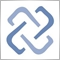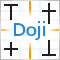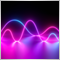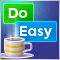Do you like the article?
Share it with others —
Use new possibilities of MetaTrader 5

#### Similar articles# Matrices and vectors in MQL59 3300Collections of ordered data, wherein all elements have the same type, are usually operated through Arrays, in which each element can be accessed by its index. Arrays are widely used in solving various linear algebra problems, in mathematical modeling tasks, in machine learning, etc. In general terms, the solution of such problems is based on mathematical operations using matrices and vectors, with which very complex transformations can be compactly written in the form of simple formulas. Programming of such operations requires good knowledge in mathematics along with the ability to write complex nested loops. Debugging and bug fixing in such programs can be quite challenging.

By using special data types 'matrix' and 'vector', it is possible to create the code which is very close to mathematical notation while avoiding the need to create nested loops or to mind correct indexing of arrays in calculations. In this article, we will see how to create, initialize, and use matrix and vector objects in MQL5.

### Type 'vector'

vector is a one-dimensional, double-type array. The following operations are defined on vectors: addition and multiplication, as well as the Norm for obtaining vector length or module. In programming, vectors are usually represented by arrays of homogeneous elements, on which no regular vector operations might be defined, i.e. arrays cannot be added or multiplied, and they have no norm.

In mathematics, vectors can be represented as row vectors, i.e. an array consisting of one row and n columns, and string vectors, i.e. a matrix of one column and n rows. In MQL5, type 'vector' does not have row and column subtypes, so the programmer must understand which vector type is used in a particular operation.

Use the following built-in methods to create and initialize vectors.

Methods
NumPy analog
Description
void vector.Init( ulong size);   Creates a vector of the specified length, in which values are undefined
static vector vector::Ones(ulong size);
ones
Creates a vector of the specified length, filled with ones
static vector vector::Zeros(ulong size);
zeros
Creates a vector of the specified length, filled with zeros
static vector vector::Full(ulong size,double value);
full
Creates a vector of the specified length, filled with the specified value
operator =

Returns a copy of the vector
void vector.Resize(const vector v);
Resizes a vector by adding new values to the end

Vector creation examples:

```void OnStart()
{
//--- vector initialization examples
vector v;
v.Init(7);
Print("v = ", v);

vector v1=vector::Ones(5);
Print("v1 = ", v1);

vector v2=vector::Zeros(3);
Print("v2 = ", v2);

vector v3=vector::Full(6, 2.5);
Print("v3 = ", v3);

vector v4{1, 2, 3};
Print("v4 = ", v4);
v4.Resize(5);
Print("after Resize(5) v4 = ", v4);

vector v5=v4;
Print("v5 = ", v5);
v4.Fill(7);
Print("v4 = ", v4, "   v5 =",v5);

}

/*
Execution result

v = [4,5,6,8,10,12,12]
v1 = [1,1,1,1,1]
v2 = [0,0,0]
v3 = [2.5,2.5,2.5,2.5,2.5,2.5]
v4 = [1,2,3]
after Resize(5) v4 = [1,2,3,7,7]
v5 = [1,2,3,7,7]
v4 = [7,7,7,7,7]   v5 =[1,2,3,7,7]

*/
```

The Init() method can be used not only to allocate memory for the vector, but also to initialize vector elements with values using a function. In this case, the vector size is passed to Init as the first parameter and the function name is passed as the second one. If the function contains parameters, these parameters should be specified immediately after the function name, separated by a comma.

The function itself must contain a reference to the vector which is passed into it as the first parameter. The vector should not be passed during Init call. Let's view the method operation using the Arange function as an example. This function mimics numpy.arange.

```void OnStart()
{
//---
vector v;
v.Init(7,Arange,10,0,0.5); // 3 parameters are passed with Arange call
Print("v = ", v);
Print("v.size = ",v.Size());
}
//+------------------------------------------------------------------+
//|  Values are generated within the half-open interval [start, stop)|
//+------------------------------------------------------------------+
void Arange(vector& v, double stop, double start = 0, double step = 1) // the function has 4 parameters
{
if(start >= stop)
{
PrintFormat("%s wrong parameters! start=%G  stop=%G", __FILE__,start, stop);
return;
}
//---
int size = (int)((stop - start) / step);
v.Resize(size);
double value = start;
for(ulong i = 0; i < v.Size(); i++)
{
v[i] = value;
value += step;
}
}

/*
Execution result

v = [0,0.5,1,1.5,2,2.5,3,3.5,4,4.5,5,5.5,6,6.5,7,7.5,8,8.5,9,9.5]
v.size = 20

*/
```

The Arange function has two optional parameters, "start" and "step". So, another possible call of Init(7,Arange,10) and the relevant result are as follows:

```//+------------------------------------------------------------------+
//| Script program start function                                    |
//+------------------------------------------------------------------+
void OnStart()
{
//---
vector v;
v.Init(7,Arange,10);
Print("v = ", v);
Print("v.size = ",v.Size());
}
...

/*

v = [0,1,2,3,4,5,6,7,8,9]
v.size = 10

*/
```

### Operations with vectors

Usual operations of addition, subtraction, multiplication and division using a scalar can be performed on vectors.

```//+------------------------------------------------------------------+
//|                                              vector2_article.mq5 |
//|                                  Copyright 2021, MetaQuotes Ltd. |
//|                                             https://www.mql5.com |
//+------------------------------------------------------------------+
#property version   "1.00"
//+------------------------------------------------------------------+
//| Script program start function                                    |
//+------------------------------------------------------------------+
void OnStart()
{
//---
vector v= {1, 2, 3, 4, 5};
Print("Examples without saving vector changes");
Print("v = ", v);
Print("v+5 = ", v+5);
Print("v-Pi= ", v-M_PI);
Print("v*2.0= ", v*2);
Print("v/3.0= ", v/3.0);

Print("Save all vector changes");
Print("v = ", v);
Print("v+5 = ", v=v+5);
Print("v-Pi= ", v=v-M_PI);
Print("v*2.0= ", v= v*2);
Print("v/3.0= ", v= v/3.0);
}
/*
Execution result

Examples without saving vector changes
v = [1,2,3,4,5]
v+5 = [6,7,8,9,10]
v-Pi= [-2.141592653589793,-1.141592653589793,-0.1415926535897931,0.8584073464102069,1.858407346410207]
v*2.0= [2,4,6,8,10]
v/3.0= [0.3333333333333333,0.6666666666666666,1,1.333333333333333,1.666666666666667]
Save all vector changes
v = [1,2,3,4,5]
v+5 = [6,7,8,9,10]
v-Pi= [2.858407346410207,3.858407346410207,4.858407346410207,5.858407346410207,6.858407346410207]
v*2.0= [5.716814692820414,7.716814692820414,9.716814692820414,11.71681469282041,13.71681469282041]
v/3.0= [1.905604897606805,2.572271564273471,3.238938230940138,3.905604897606805,4.572271564273471]

*/
//+------------------------------------------------------------------+

```

Vectors support element-wise operations of addition, subtraction, multiplication and division of two same-sized vectors.

```void OnStart()
{
//---
vector a = {1, 2, 3};
vector b = {2, 4, 6};
Print("a + b = ", a + b);
Print("a - b = ", a - b);
Print("a * b = ", a * b);
Print("b / a = ", b / a);
}

/*
Execution result

a + b = [3,6,9]
a - b = [-1,-2,-3]
a * b = [2,8,18]
b / a = [2,2,2]

*/
```

Four product operations are defined for this data type.

```void OnStart()
{
//---
vector a={1, 2, 3};
vector b={4, 5, 6};
Print("a = ", a);
Print("b = ", b);
Print("1) a.Dot(b) = ", a.Dot(b));
Print("2) a.MatMul(b) = ", a.MatMul(b));
Print("3) a.Kron(b) = ", a.Kron(b));
Print("4) a.Outer(b) = \n", a.Outer(b));
}
/*
Execution result

a = [1,2,3]
b = [4,5,6]
1) a.Dot(b) = 32.0
2) a.MatMul(b) = 32.0
3) a.Kron(b) = [[4,5,6,8,10,12,12,15,18]]
4) a.Outer(b) =
[[4,5,6]
[8,10,12]
[12,15,18]]

*/
```

As you can see from the example, the Outer method returns a matrix in which the number of rows and columns correspond to the sizes of the multiplied vectors. The Dot and MatMul operate the same way.

### Vector norm

Vector and matrix norm represents the vector length (magnitude) and absolute value. Three possible ways to calculate the norm of a vector are listed in ENUM_VECTOR_NORM.
```void OnStart()
{
//---
struct str_vector_norm
{
ENUM_VECTOR_NORM  norm;
int               value;
};
str_vector_norm vector_norm[]=
{
{VECTOR_NORM_INF,       0},
{VECTOR_NORM_MINUS_INF, 0},
{VECTOR_NORM_P,         0},
{VECTOR_NORM_P,         1},
{VECTOR_NORM_P,         2},
{VECTOR_NORM_P,         3},
{VECTOR_NORM_P,         4},
{VECTOR_NORM_P,         5},
{VECTOR_NORM_P,         6},
{VECTOR_NORM_P,         7},
{VECTOR_NORM_P,        -1},
{VECTOR_NORM_P,        -2},
{VECTOR_NORM_P,        -3},
{VECTOR_NORM_P,        -4},
{VECTOR_NORM_P,        -5},
{VECTOR_NORM_P,        -6},
{VECTOR_NORM_P,        -7}
};
vector v{1, 2, 3, 4, 5, 6, 7};
double norm;
Print("v = ", v);
//---
for(int i=0; i<ArraySize(vector_norm); i++)
{
switch(vector_norm[i].norm)
{
case VECTOR_NORM_INF :
norm=v.Norm(VECTOR_NORM_INF);
Print("v.Norm(VECTOR_NORM_INF) = ", norm);
break;
case VECTOR_NORM_MINUS_INF :
norm=v.Norm(VECTOR_NORM_MINUS_INF);
Print("v.Norm(VECTOR_NORM_MINUS_INF) = ", norm);
break;
case VECTOR_NORM_P :
norm=v.Norm(VECTOR_NORM_P, vector_norm[i].value);
PrintFormat("v.Norm(VECTOR_NORM_P,%d) = %G", vector_norm[i].value, norm);
}
}
}
/*

v = [1,2,3,4,5,6,7]
v.Norm(VECTOR_NORM_INF) = 7.0
v.Norm(VECTOR_NORM_MINUS_INF) = 1.0
v.Norm(VECTOR_NORM_P,0) = 7
v.Norm(VECTOR_NORM_P,1) = 28
v.Norm(VECTOR_NORM_P,2) = 11.8322
v.Norm(VECTOR_NORM_P,3) = 9.22087
v.Norm(VECTOR_NORM_P,4) = 8.2693
v.Norm(VECTOR_NORM_P,5) = 7.80735
v.Norm(VECTOR_NORM_P,6) = 7.5473
v.Norm(VECTOR_NORM_P,7) = 7.38704
v.Norm(VECTOR_NORM_P,-1) = 0.385675
v.Norm(VECTOR_NORM_P,-2) = 0.813305
v.Norm(VECTOR_NORM_P,-3) = 0.942818
v.Norm(VECTOR_NORM_P,-4) = 0.980594
v.Norm(VECTOR_NORM_P,-5) = 0.992789
v.Norm(VECTOR_NORM_P,-6) = 0.99714
v.Norm(VECTOR_NORM_P,-7) = 0.998813

*/
```

Using the norm, you can measure the distance between two vectors:

```void OnStart()
{
//---
vector a{1,2,3};
vector b{2,3,4};
double distance=(b-a).Norm(VECTOR_NORM_P,2);
Print("a = ",a);
Print("b = ",b);
Print("|a-b| = ",distance);
}
/*
Execution result

a = [1,2,3]
b = [2,3,4]
|a-b| = 1.7320508075688772

*/
```

### Type 'matrix'

The vector is a special case of a matrix, which is actually a two-dimensional array of type double. Thus, a matrix can be considered as an array of same-sized vectors. The number of matrix rows corresponds to the number of vectors, while the number of columns is equal to vector length.

The operations of addition and multiplication are also available for matrices. Conventional programming languages use arrays to represent matrices. However, regular arrays cannot be added or multiplied by each other, and they do not have the norm. Mathematics considers many different matrix types. For example, identity matrix, symmetric, skew-symmetric, upper and lower triangular matrices, and other types.

A matrix can be created and initialized using built-in methods similar to vector methods.

Method

Analogous method in NumPy

Description

void static matrix.Eye(const int rows, const int cols, const int ndiag=0)

eye

Constructs a matrix with ones on a specified diagonal and zeros elsewhere

void matrix.Identity()

identity

Fills a matrix with ones on the main diagonal and zeros elsewhere

void static matrix.Ones(const int rows, const int cols)

ones

Constructs a new matrix by the number of rows and columns, filled with ones

void static matrix.Zeros(const int rows, const int cols)

zeros

Constructs a new matrix by the number of rows and columns, filled with zeros

void static matrix.Tri(const int rows, const int cols, const int ndiag=0)
tri  Constructs a matrix with ones on a specified diagonal and below and zeros elsewhere
void matrix.Diag(const vector v, const int ndiag=0)  diag  Extracts a diagonal or constructs a diagonal matrix

void matrix.Full(const int rows, const int cols, const scalar value)

full

Constructs a new matrix by the number of rows and columns, filled with a scalar value

void matrix.Fill(const scalar value)     Fills the matrix with the specified value

Matrix construction and filling examples:

```void OnStart()
{
//---
matrix m{{1, 2, 3}, {4, 5, 6}, {7, 8, 9}};
Print("m = \n", m);
matrix ones=matrix::Ones(4, 4);
Print("ones = \n", ones);
matrix zeros=matrix::Zeros(4, 4);
Print("zeros = \n", zeros);
matrix eye=matrix::Eye(4, 4);
Print("eye = \n", eye);

matrix identity(4, 5);
Print("matrix_identity\n", identity);
identity.Identity();
Print("matrix_identity\n", identity);

matrix tri=matrix::Tri(3, 4);
Print("tri = \n", tri);
Print("tri.Transpose() = \n", tri.Transpose()); // transpose the matrix

matrix diag(5, 5);
Print("diag = \n", diag);
vector d{1, 2, 3, 4, 5};
diag.Diag(d);
Print("diag = \n", diag); // insert values from the vector into the matrix diagonal

matrix fill(5, 5);
fill.Fill(10);
Print("fill = \n", fill);

matrix full =matrix::Full(5, 5, 100);
Print("full = \n", full);

matrix init(5, 7);
Print("init = \n", init);
m.Init(4, 6);
Print("init = \n", init);

matrix resize=matrix::Full(2, 2, 5);
resize.Resize(5,5);
Print("resize = \n", resize);
}
/*
Execution result

m =
[[1,2,3]
[4,5,6]
[7,8,9]]
ones =
[[1,1,1,1]
[1,1,1,1]
[1,1,1,1]
[1,1,1,1]]
zeros =
[[0,0,0,0]
[0,0,0,0]
[0,0,0,0]
[0,0,0,0]]
eye =
[[1,0,0,0]
[0,1,0,0]
[0,0,1,0]
[0,0,0,1]]
matrix_identity
[[1,0,0,0,0]
[0,1,0,0,0]
[0,0,1,0,0]
[0,0,0,1,0]]
matrix_identity
[[1,0,0,0,0]
[0,1,0,0,0]
[0,0,1,0,0]
[0,0,0,1,0]]
tri =
[[1,0,0,0]
[1,1,0,0]
[1,1,1,0]]
tri.Transpose() =
[[1,1,1]
[0,1,1]
[0,0,1]
[0,0,0]]
diag =
[[0,0,0,0,0]
[0,0,0,0,0]
[0,0,0,0,0]
[0,0,0,0,0]
[0,0,0,0,0]]
diag =
[[1,0,0,0,0]
[0,2,0,0,0]
[0,0,3,0,0]
[0,0,0,4,0]
[0,0,0,0,5]]
fill =
[[10,10,10,10,10]
[10,10,10,10,10]
[10,10,10,10,10]
[10,10,10,10,10]
[10,10,10,10,10]]
full =
[[100,100,100,100,100]
[100,100,100,100,100]
[100,100,100,100,100]
[100,100,100,100,100]
[100,100,100,100,100]]
resize =
[[5,5,0,0,0]
[5,5,0,0,0]
[0,0,0,0,0]
[0,0,0,0,0]
[0,0,0,0,0]]

*/

```

The following example shows how you can use custom functions when filling matrices:

```//+------------------------------------------------------------------+
//| Script program start function                                    |
//+------------------------------------------------------------------+
void OnStart()
{
//---
matrix random(4, 5, MatrixRandom);
Print("random = \n",random);

matrix init(3, 6, MatrixSetValues);
Print("init = \n", init);

}
//+------------------------------------------------------------------+
//| Fills the matrix with random values                              |
//+------------------------------------------------------------------+
void MatrixRandom(matrix& m)
{
for(ulong r=0; r<m.Rows(); r++)
{
for(ulong c=0; c<m.Cols(); c++)
{
m[r][c]=double(MathRand())/32767.;
}
}
}
//+------------------------------------------------------------------+
//| Fills the matrix with powers of a number                         |
//+------------------------------------------------------------------+
void MatrixSetValues(matrix& m, double initial=1)
{
double value=initial;
for(ulong r=0; r<m.Rows(); r++)
{
for(ulong c=0; c<m.Cols(); c++)
{
m[r][c]=value;
value*=2;
}
}
}

/*
Execution result

random =
[[0.4200262459181494,0.5014496292001098,0.7520371105075229,0.652058473464156,0.08783227027191992]
[0.5991088595233008,0.4311960203863643,0.8718832972197638,0.1350138859218116,0.901882992034669]
[0.4964445936460463,0.8354747154148991,0.5258339182714317,0.6055482650227363,0.5952940458388012]
[0.3959166234321116,0.8146916104617451,0.2053590502639851,0.2657551805169835,0.3672292245246742]]
init =
[[1,2,4,8,16,32]
[64,128,256,512,1024,2048]
[4096,8192,16384,32768,65536,131072]]

*/
```

A matrix can be constructed without value initialization in two ways:

```//--- create a matrix of a given 'rows x cols' size
matrix m(3, 3);

// ------ equivalent
matrix m;
m.Resize(3, 3);
```

Matrix norm

The nine ways to calculate a matrix norm are listed in ENUM_MATRIX_NORM.

```void OnStart()
{
//---
ENUM_MATRIX_NORM matrix_norm[]= {MATRIX_NORM_FROBENIUS,
MATRIX_NORM_SPECTRAL,
MATRIX_NORM_NUCLEAR,
MATRIX_NORM_INF,
MATRIX_NORM_MINUS_INF,
MATRIX_NORM_P1,
MATRIX_NORM_MINUS_P1,
MATRIX_NORM_P2,
MATRIX_NORM_MINUS_P2
};
matrix m{{1,2,3},{4,5,6},{7,8,9}};
Print("matrix m:\n",m);
//--- compute the norm using all ways
double norm;
for(int i=0; i<ArraySize(matrix_norm); i++)
{
norm=m.Norm(matrix_norm[i]);
PrintFormat("%d. Norm(%s) = %.6f",i+1, EnumToString(matrix_norm[i]),norm);
}
//---
return;
}

/*
Execution result

matrix m:
[[1,2,3]
[4,5,6]
[7,8,9]]
1. Norm(MATRIX_NORM_FROBENIUS) = 16.881943
2. Norm(MATRIX_NORM_SPECTRAL) = 14.790157
3. Norm(MATRIX_NORM_NUCLEAR) = 17.916473
4. Norm(MATRIX_NORM_INF) = 24.000000
5. Norm(MATRIX_NORM_MINUS_INF) = 6.000000
6. Norm(MATRIX_NORM_P1) = 18.000000
7. Norm(MATRIX_NORM_MINUS_P1) = 12.000000
8. Norm(MATRIX_NORM_P2) = 16.848103
9. Norm(MATRIX_NORM_MINUS_P2) = 0.000000

*/
```

### Operations with matrices and vectors

Matrices provide special methods for solving mathematical problems:

• Transposition
• Element-wise matrix addition, subtraction, multiplication and division
• Addition, subtraction, multiplication and division of matrix elements by a scalar
• Product of matrices and vectors by the MatMul method (matrix product)
• Inner()
• Outer()
• Kron()
• Inv() — matrix inverse
• Solve() — solve a system of linear equations
• LstSq() — return the least-squares solution of linear algebraic equations (for non-square or degenerate matrices)
• PInv() — pseudo-inverse least squares matrix
• Operations with columns, rows, and diagonals

Matrix decomposition:

Method

Analogous method in NumPy

Description
bool matrix.Cholesky(matrix& L) cholesky Computes the Cholesky decomposition
bool matrix.QR(matrix& Q, matrix& R) qr

Computes the QR decomposition

bool matrix.SVD(matrix& U, matrix& V, vector& singular_values)

svd

Computes the SVD decomposition

bool matrix.Eig(matrix& eigen_vectors, vector& eigen_values)

eig

Computes the eigenvalues and right eigenvectors of a square matrix

bool matrix.EigVals(vector& eigen_values)

eigvals

Computes the eigenvalues of a general matrix

bool matrix.LU(matrix& L, matrix& U)

Implements an LU decomposition of a matrix: the product of a lower triangular matrix and an upper triangular matrix

bool matrix.LUP(matrix& L, matrix& U, matrix& P)

Implements a LUP decomposition with partial pivoting, which is an LU factorization with a permutation of rows: PA = LU

### Product of matrices and vectors

The MatMul() is defined to compute the matrix product of matrices and vectors. This method is often used in solving various mathematical problems. The following two options are possible when multiplying a matrix and a vector:

• The horizontal vector on the left is multiplied by the matrix on the right; the vector length is equal to the number of columns in the matrix;
• The matrix on the left is multiplied by the vertical vector on the right; the number of the matrix columns is equal to the vector length.

If the vector length is not equal to the number of columns in the matrix, a critical execution error will be generated.

To multiply two matrices, their form should be as follows: A[M,N] * B[N,K] = C[M,K], i.e. the number of columns in the matrix on the left must be equal to the number of rows in the matrix on the right. If the dimensions are not consistent, the result is an empty matrix. Let's view all matrix product variants with examples.

```void OnStart()
{
//--- initialize matrices
matrix m35, m52;
m35.Init(3,5,Arange);
m52.Init(5,2,Arange);
//---
Print("1. Product of horizontal vector v and matrix m[3,5]");
vector v3 = {1,2,3};
Print("On the left v3 = ",v3);
Print("On the right m35 = \n",m35);
Print("v3.MatMul(m35) = horizontal vector v \n",v3.MatMul(m35));
//--- show that this is really a horizontal vector
Print("\n2. Product of matrix m[1,3] and matrix m[3,5]");
matrix m13;
m13.Init(1,3,Arange,1);
Print("On the left m13 = \n",m13);
Print("On the right m35 = \n",m35);
Print("m13.MatMul(m35) = matrix m[1,5] \n",m13.MatMul(m35));

Print("\n3. Product of matrix m[3,5] and vertical vector v");
vector v5 = {1,2,3,4,5};
Print("On the left m35 = \n",m35);
Print("On the right v5 = ",v5);
Print("m35.MatMul(v5) = vertical vector v \n",m35.MatMul(v5));
//--- show that this is really a vertical vector
Print("\n4. Product of matrix m[3,5] and matrix m[5,1]");
matrix m51;
m51.Init(5,1,Arange,1);
Print("On the left m35 = \n",m35);
Print("On the right m51 = \n",m51);
Print("m35.MatMul(m51) = matrix v \n",m35.MatMul(m51));

Print("\n5. Product of matrix m[3,5] and matrix m[5,2]");
Print("On the left m35 = \n",m35);
Print("On the right m52 = \n",m52);
Print("m35.MatMul(m52) = matrix m[3,2] \n",m35.MatMul(m52));

Print("\n6. Product of horizontal vector v and matrix m[5,2]");
Print("On the left v5 = \n",v5);
Print("On the right m52 = \n",m52);
Print("v5.MatMul(m52) = horizontal vector v \n",v5.MatMul(m52));

Print("\n7. Outer() product of horizontal vector v and vertical vector v");
Print("On the left v5 = \n",v5);
Print("On the right v3 = \n",v3);
Print("v5.Outer(v3) = matrix m[5,3] \n",v5.Outer(v3));
//--- show that the product of matrices generates the same result
Print("\n8. Outer() product of the matrix m[1,5] and matrix m[3,1]");
matrix m15,m31;
m15.Init(1,5,Arange,1);
m31.Init(3,1,Arange,1);
Print("On the left m[1,5] = \n",m15);
Print("On the right m31 = \n",m31);
Print("m15.Outer(m31) = matrix m[5,3] \n",m15.Outer(m31));
}
//+------------------------------------------------------------------+
//|  Fill the matrix with increasing values                          |
//+------------------------------------------------------------------+
void Arange(matrix & m, double start = 0, double step = 1) // the function has three parameters
{
//---
ulong cols = m.Cols();
ulong rows = m.Rows();
double value = start;
for(ulong r = 0; r < rows; r++)
{
for(ulong c = 0; c < cols; c++)
{
m[r][c] = value;
value += step;
}
}
//---
}
/*
Execution result

1. Product of horizontal vector v and matrix m[3,5]
On the left v3 = [1,2,3]
On the right m35 =
[[0,1,2,3,4]
[5,6,7,8,9]
[10,11,12,13,14]]
v3.MatMul(m35) = horizontal vector v
[40,46,52,58,64]

2. Product of matrix m[1,3] and matrix m[3,5]
On the left m13 =
[[1,2,3]]
On the right m35 =
[[0,1,2,3,4]
[5,6,7,8,9]
[10,11,12,13,14]]
m13.MatMul(m35) = matrix m[1,5]
[[40,46,52,58,64]]

3. Product of matrix m[3,5] and vertical vector v
On the left m35 =
[[0,1,2,3,4]
[5,6,7,8,9]
[10,11,12,13,14]]
On the right v5 = [1,2,3,4,5]
m35.MatMul(v5) = vertical vector v
[40,115,190]

4. Product of matrix m[3,5] and matrix m[5,1]
On the left m35 =
[[0,1,2,3,4]
[5,6,7,8,9]
[10,11,12,13,14]]
On the right m51 =
[



]
m35.MatMul(m51) = matrix v
[

]

5. Product of matrix m[3,5] and matrix m[5,2]
On the left m35 =
[[0,1,2,3,4]
[5,6,7,8,9]
[10,11,12,13,14]]
On the right m52 =
[[0,1]
[2,3]
[4,5]
[6,7]
[8,9]]
m35.MatMul(m52) = matrix m[3,2]
[[60,70]
[160,195]
[260,320]]

6. The product of horizontal vector v and matrix m[5,2]
On the left v5 =
[1,2,3,4,5]
On the right m52 =
[[0,1]
[2,3]
[4,5]
[6,7]
[8,9]]
v5.MatMul(m52) = horizontal vector v
[80,95]

7. Outer() product of horizontal vector v and vertical vector v
On the left v5 =
[1,2,3,4,5]
On the right v3 =
[1,2,3]
v5.Outer(v3) = matrix m[5,3]
[[1,2,3]
[2,4,6]
[3,6,9]
[4,8,12]
[5,10,15]]

8. Outer() product of the matrix m[1,5] and matrix m[3,1]
On the left m[1,5] =
[[1,2,3,4,5]]
On the right m31 =
[

]
m15.Outer(m31) = matrix m[5,3]
[[1,2,3]
[2,4,6]
[3,6,9]
[4,8,12]
[5,10,15]]

*/
```

For a better understanding of how matrix and vector types are arranged, these examples show how matrices can be used instead of vectors. It means that vectors can be represented as matrices.

### Complex numbers of type 'complex'

Some mathematical problems require the use of the new data type 'complex numbers'. Type complex is a structure:
```struct complex
{
double             real;   // real part
double             imag;   // imaginary part
};
```
The 'complex' type can be passed by value as a parameter for MQL5 functions (in contrast to ordinary structures, which are only passed by reference). For functions imported from DLLs, the 'complex' type must be passed only by reference.

The 'i' suffix is used to describe complex constants:
```complex square(complex c)
{
return(c*c);
}

void OnStart()
{
Print(square(1+2i));  // a constant is passed as a parameter
}

// will print "(-3,4)" - a string representation of a complex number
```
Only simple operations are available for complex numbers: =, +, -, *, /, +=, -=, *=, /=, ==, !=.

Support for additional mathematical functions will be added soon, enabling the calculation of the absolute value, sine, cosine and others

Translated from Russian by MetaQuotes Ltd.
Original article: https://www.mql5.com/ru/articles/9805

#### Other articles by this authorImproved candlestick pattern recognition illustrated by the example of Doji
How to find more candlestick patterns than usual? Behind the simplicity of candlestick patterns, there is also a serious drawback, which can be eliminated by using the significantly increased capabilities of modern trading automation tools.Combinatorics and probability for trading (Part V): Curve analysis
In this article, I decided to conduct a study related to the possibility of reducing multiple states to double-state systems. The main purpose of the article is to analyze and to come to useful conclusions that may help in the further development of scalable trading algorithms based on the probability theory. Of course, this topic involves mathematics. However, given the experience of previous articles, I see that generalized information is more useful than details.Graphics in DoEasy library (Part 91): Standard graphical object events. Object name change history
In this article, I will refine the basic functionality for providing control over graphical object events from a library-based program. I will start from implementing the functionality for storing the graphical object change history using the "Object name" property as an example.Graphics in DoEasy library (Part 90): Standard graphical object events. Basic functionality
In this article, I will implement the basic functionality for tracking standard graphical object events. I will start from a double click event on a graphical object.